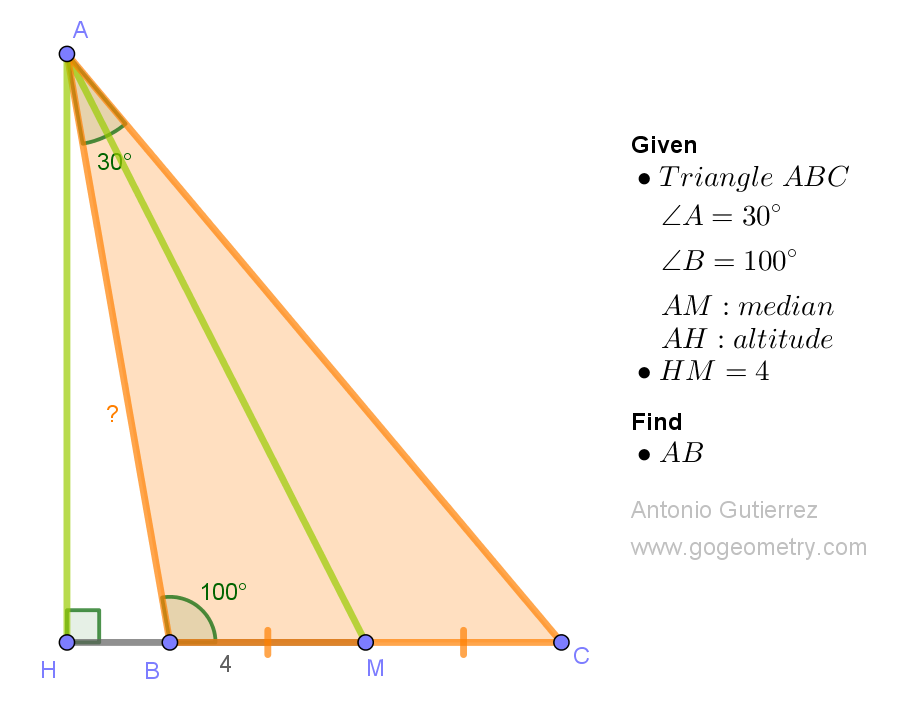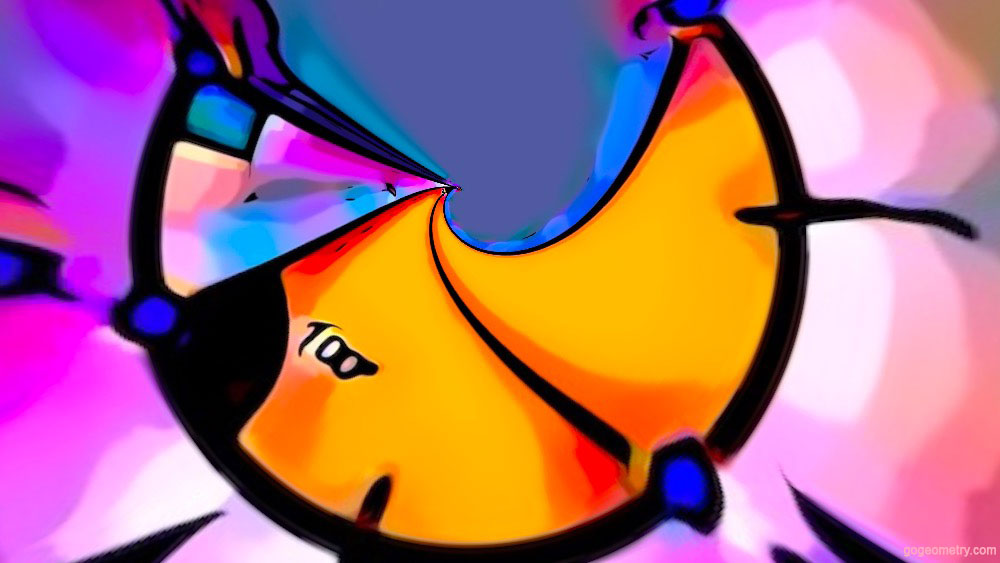# Clever Geometry Problem 1554: Finding the Length of Side AB in Triangle ABC

In triangle ABC, where angles A and B measure 100 degrees and 30 degrees, respectively, an altitude AH and a median AM are constructed. Given that the length of HM is 4 units, find the length of side AB.In triangle's embrace,
Angles defined, paths converge,
Altitude, median,
HM's secret unveils true,
AB's length, math's gift we pursue.

## Utilizing Stereographic Projection in Geometric Art: Addressing Issue 1554In this illustration, stereographic projection and mathematical transformations are utilized to distort and reshape the geometry figure of problem 1554, producing distinctive spherical effects. This exemplifies an engaging application of geometry in digital image editing, empowering users to create visually captivating images from their figures.

Stereographic projection is a mathematical technique that maps points on a sphere to points on a plane (flat surface).

#### Problem 1551